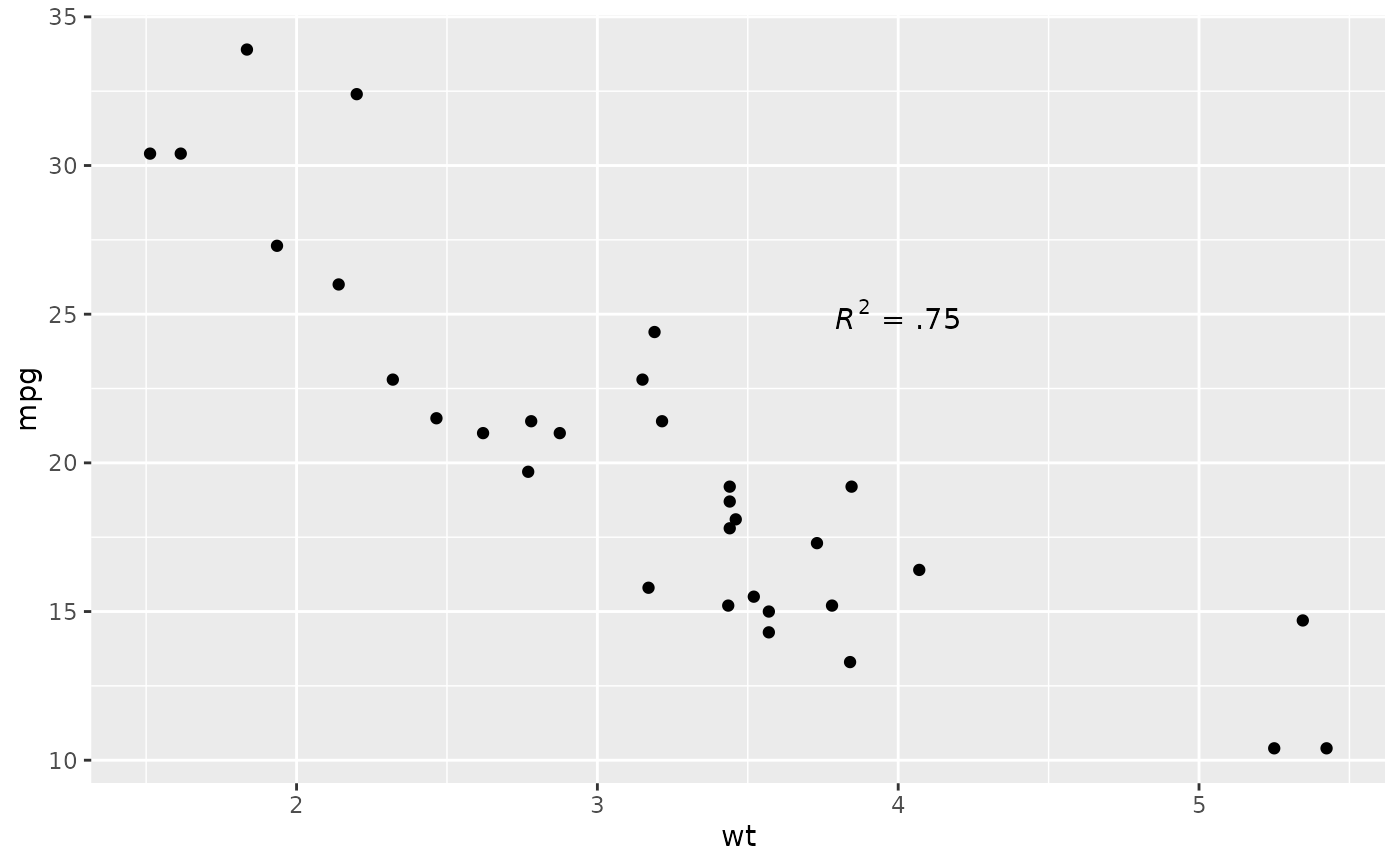This function adds geoms to a plot, but unlike a typical geom function, the properties of the geoms are not mapped from variables of a data frame, but are instead passed in as vectors. This is useful for adding small annotations (such as text labels) or if you have your data in vectors, and for some reason don't want to put them in a data frame.

## Usage

annotate(
geom,
x = NULL,
y = NULL,
xmin = NULL,
xmax = NULL,
ymin = NULL,
ymax = NULL,
xend = NULL,
yend = NULL,
...,
na.rm = FALSE
)

## Arguments

geom

name of geom to use for annotation

x, y, xmin, ymin, xmax, ymax, xend, yend

positioning aesthetics - you must specify at least one of these.

...

Other arguments passed on to layer(). These are often aesthetics, used to set an aesthetic to a fixed value, like colour = "red" or size = 3. They may also be parameters to the paired geom/stat.

na.rm

If FALSE, the default, missing values are removed with a warning. If TRUE, missing values are silently removed.

## Details

Note that all position aesthetics are scaled (i.e. they will expand the limits of the plot so they are visible), but all other aesthetics are set. This means that layers created with this function will never affect the legend.

## Unsupported geoms

Due to their special nature, reference line geoms geom_abline(), geom_hline(), and geom_vline() can't be used with annotate(). You can use these geoms directory for annotations.

## Examples

p <- ggplot(mtcars, aes(x = wt, y = mpg)) + geom_point()
p + annotate("text", x = 4, y = 25, label = "Some text")p + annotate("text", x = 2:5, y = 25, label = "Some text")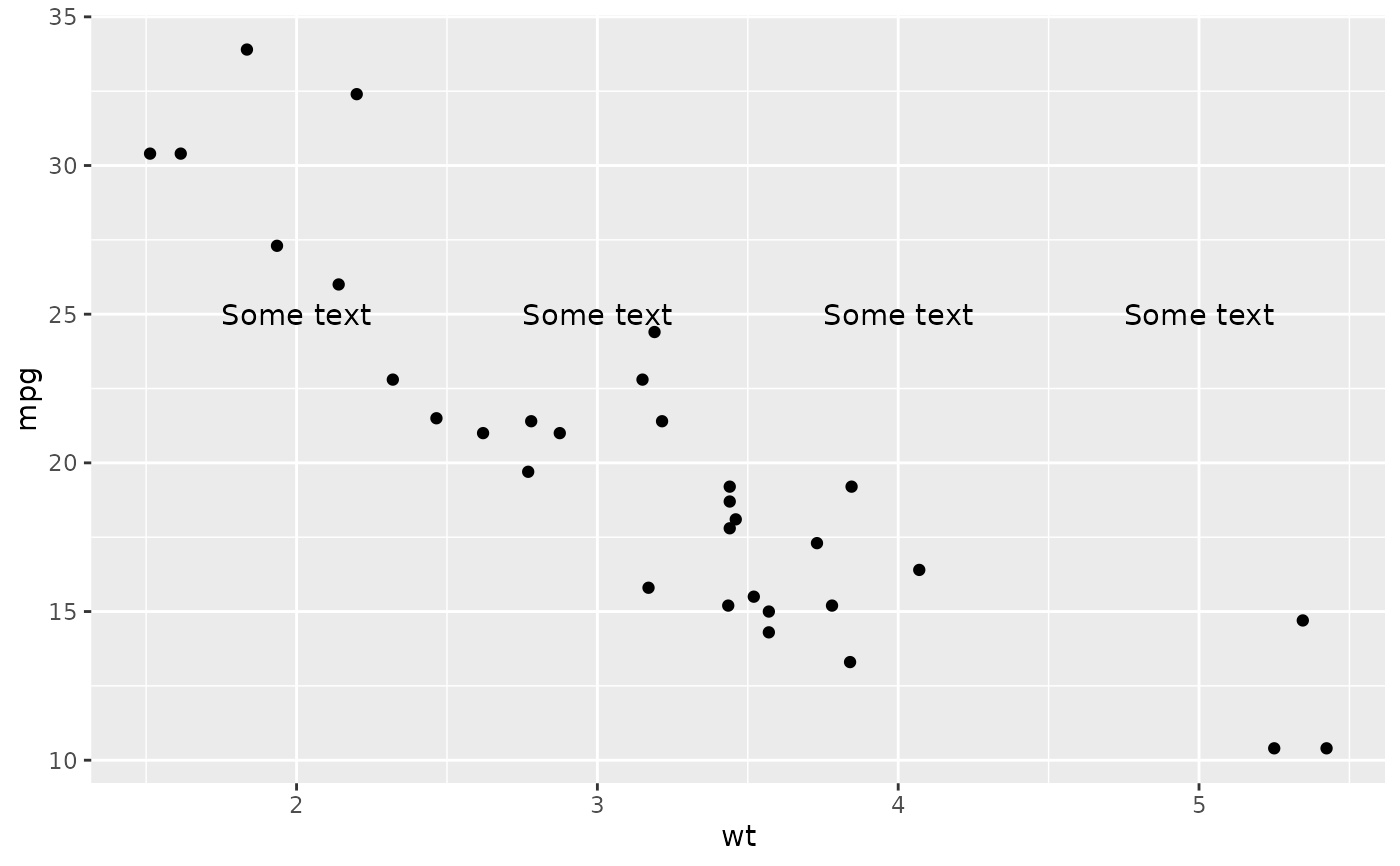p + annotate("rect", xmin = 3, xmax = 4.2, ymin = 12, ymax = 21,
alpha = .2)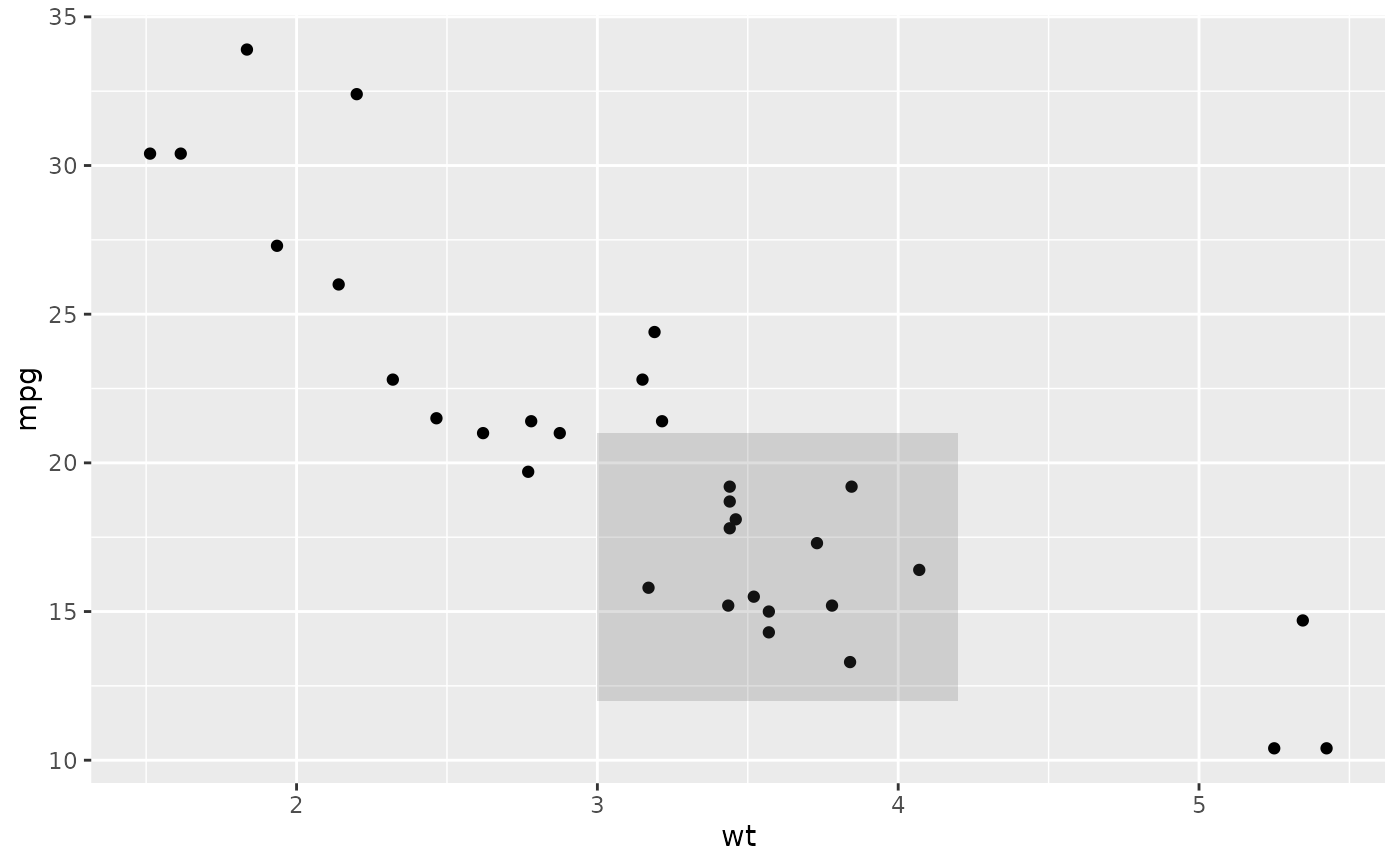p + annotate("segment", x = 2.5, xend = 4, y = 15, yend = 25,
colour = "blue")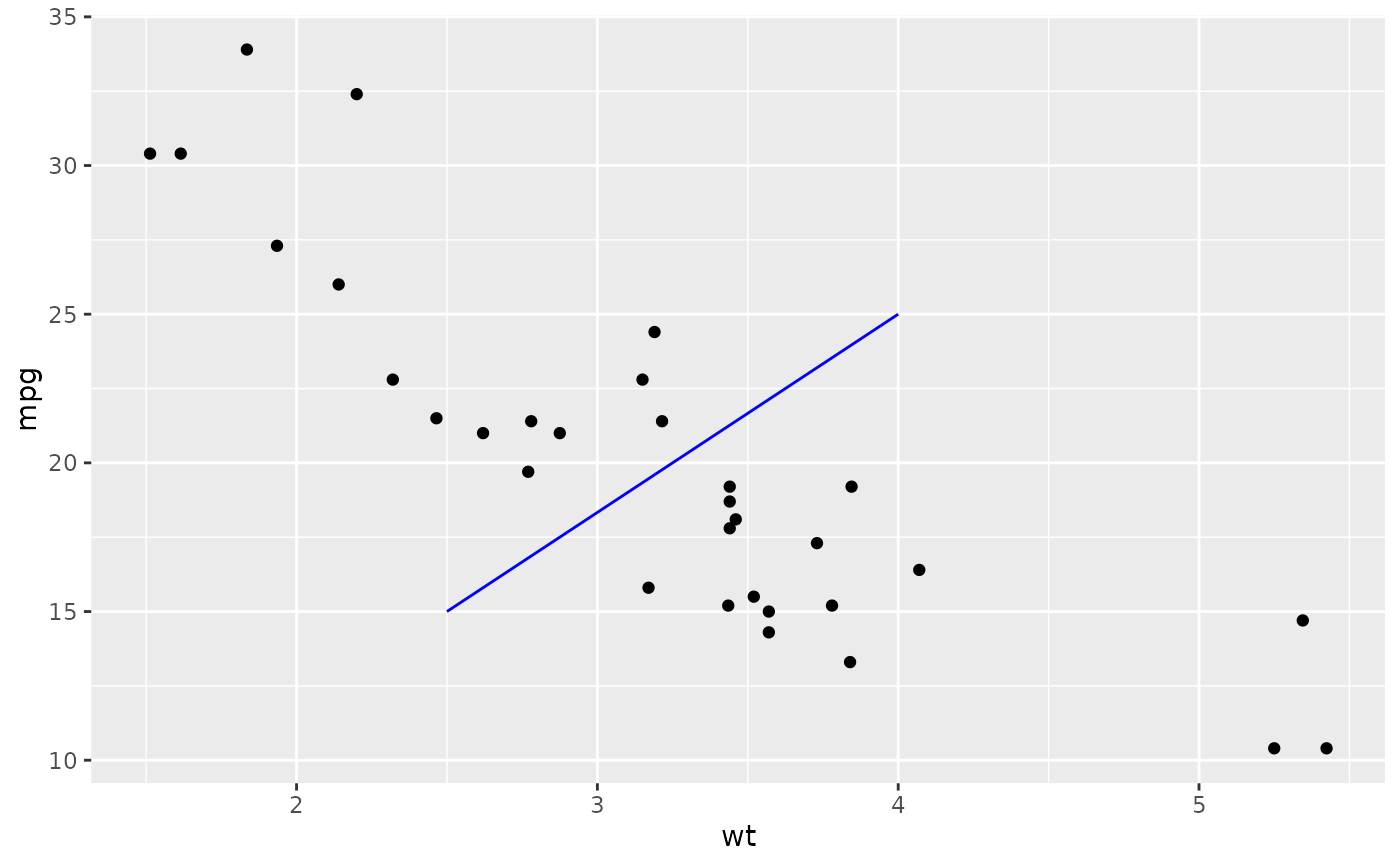p + annotate("pointrange", x = 3.5, y = 20, ymin = 12, ymax = 28,
colour = "red", size = 2.5, linewidth = 1.5)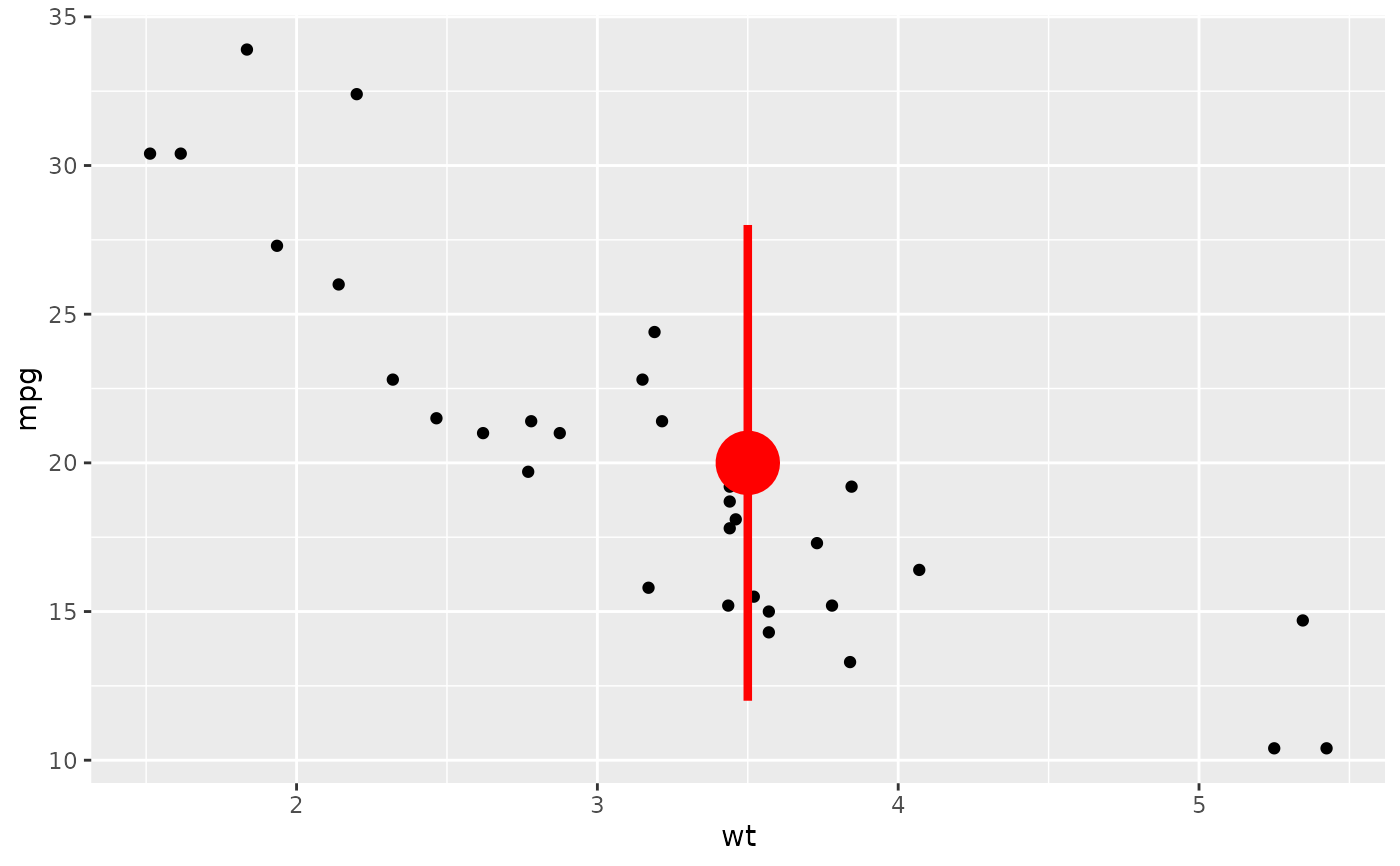p + annotate("text", x = 2:3, y = 20:21, label = c("my label", "label 2"))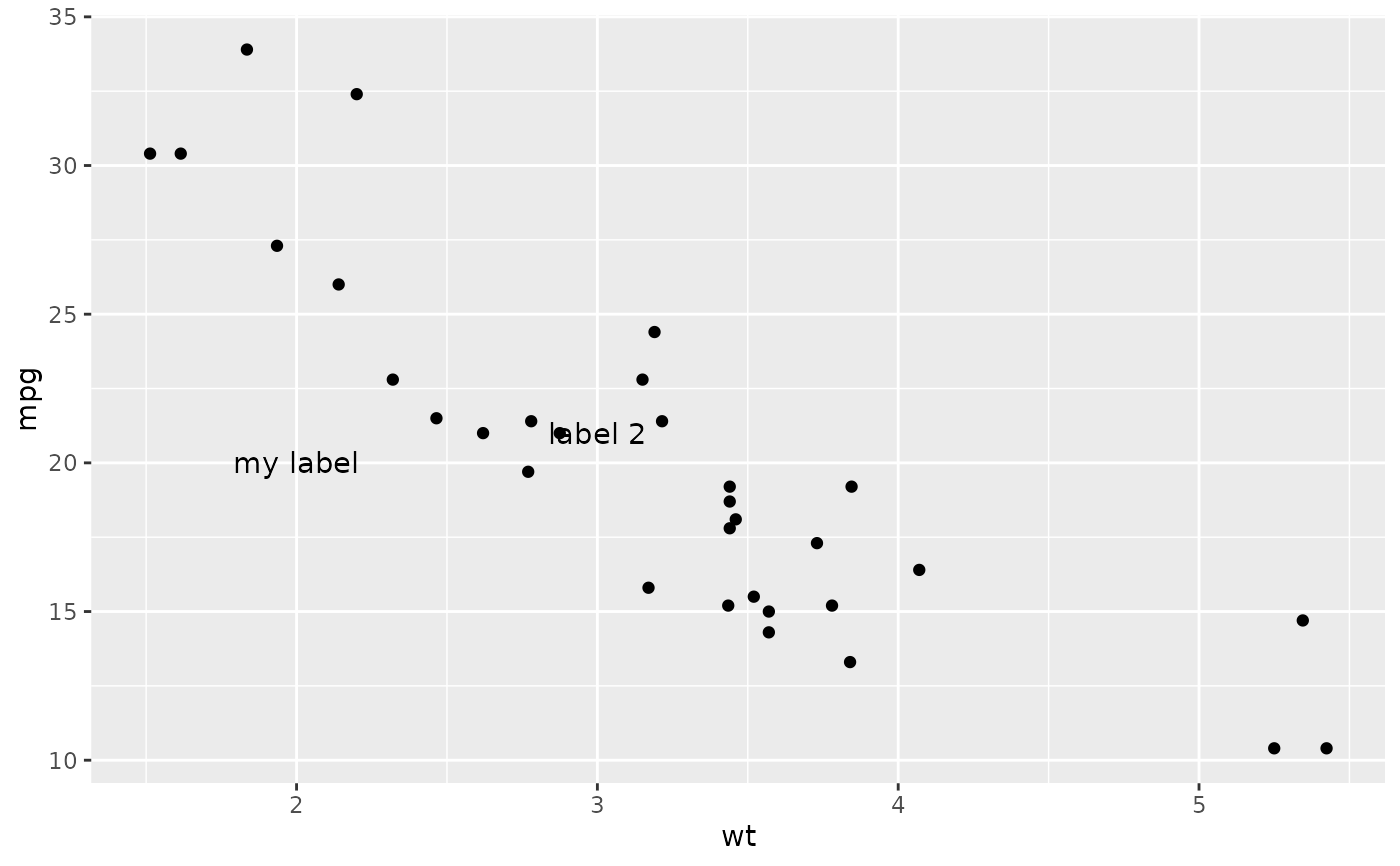p + annotate("text", x = 4, y = 25, label = "italic(R) ^ 2 == 0.75",
parse = TRUE)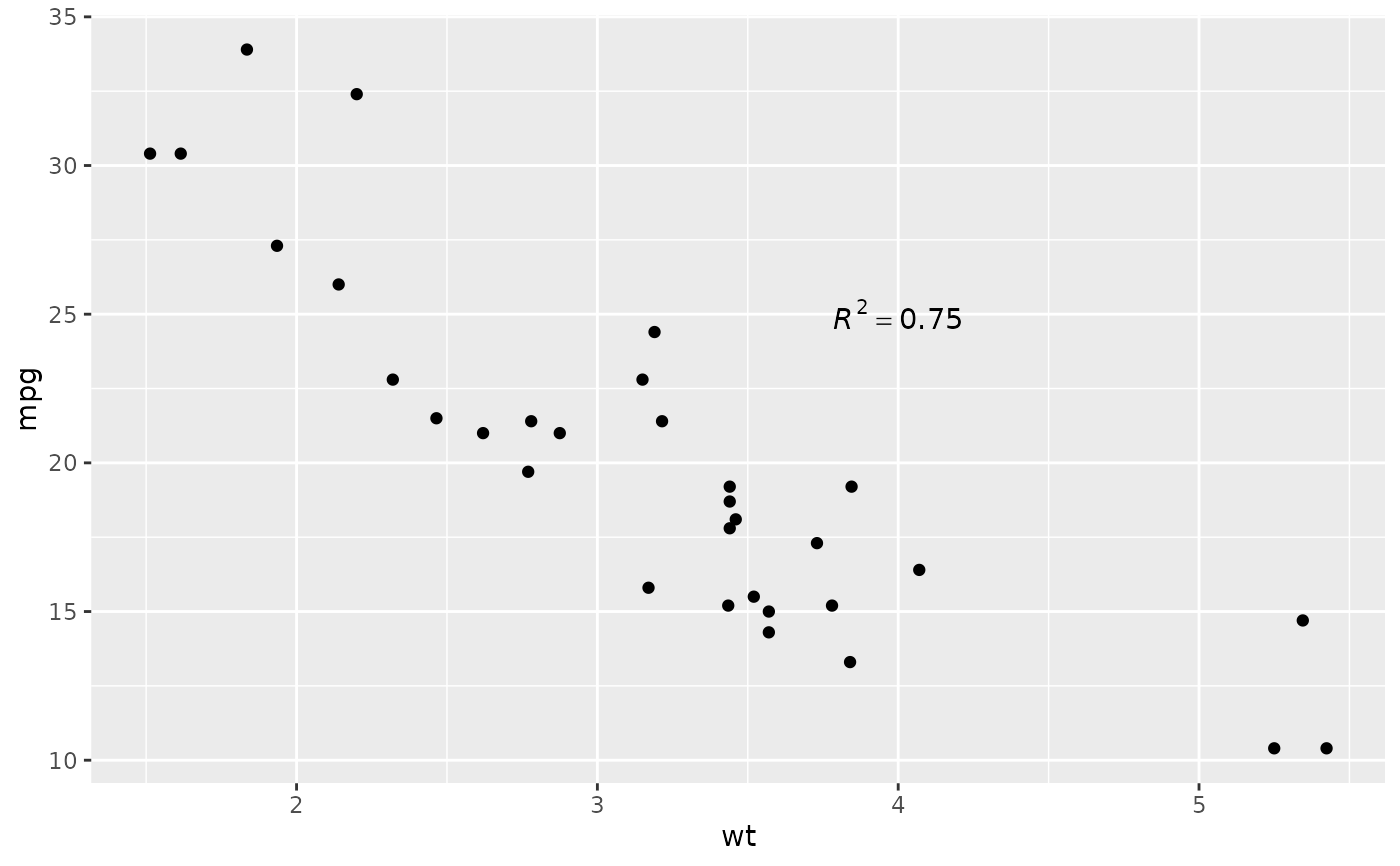p + annotate("text", x = 4, y = 25,
label = "paste(italic(R) ^ 2, \" = .75\")", parse = TRUE)